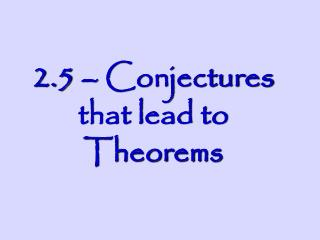Download Presentation2.5 – Conjectures that lead to Theorems

# 2.5 – Conjectures that lead to Theorems

Download Presentation## 2.5 – Conjectures that lead to Theorems

- - - - - - - - - - - - - - - - - - - - - - - - - - - E N D - - - - - - - - - - - - - - - - - - - - - - - - - - -
##### Presentation Transcript

1. 2.5 – Conjectures that lead to Theorems

2. REMEMBER? • Theorem • A statement that has been proven to be true (using deduction) • Proof • A convincing argument (using postulates, theorems and definitions) that something is true

3. PROOFS • Any definition, postulate or theorem that we learn (or have learned) is able to be used in a proof!

4. Theorems • Vertical Angles • The opposite angles formed by two intersecting lines • Vertical Angle Theorem • If two angles form a pair of vertical angles, then they are congruent

5. What do I do? • The question you need to ask at this point is 1: do the angles add up to 180°? OR 2: are the angles congruent? Then, do they want to know “x” or the angle or both?

6. Case 1 They are equal because they are vertical (2x+70)° (7x-55)°

7. Answers (7x-55)° = (2x+70)° 5x=125 x=25 The angles would both be 7(25)-55=2(25)+70= 120° each

8. Case 2 • They add up to 180° because they are a linear pair (2x+70)° (2x+10)°

9. Answers (2x+10)° + (2x+70)° = 180° 4x+80 = 180 4x = 100 X = 25 But what are the angles?

10. One of them is 2(25)+10 = 60° And the other is 2(25)+70 = 120° Because I can plug in 25 for x.